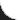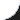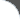Home | Tutorials | Articles | Videos | Products | Tools | Search
 Interviews | Open Source | Tag Cloud | Follow Us | Bookmark | Contact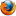In Browser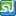StumbleUpon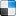del.icio.usGoogle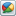Google Buzz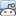redditLinkedInFacebook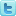TwitterLinkedinE-Mail
 Articles > Java > Coding Examples > Factorial of a number

# Factorial of a number

Find the factorial of a given number.

 File Name  : com/bethecoder/tutorials/coding/examples/FactorialTest.java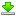Author  : Sudhakar KV Email  : [email protected]

 ``` package com.bethecoder.tutorials.coding.examples; public class FactorialTest {   /**    * @param args    */   public static void main(String[] args) {          for (int i = 0 ; i < 10 ; i ++) {       System.out.println("Factorila of '" + i + "' is " + factorial(i));     }          for (int i = 0 ; i < 10 ; i ++) {       System.out.println("Factorila of '" + i + "' is " + factorialRecursive(i));     }   }   private static int factorial(int num) {     int fact = 1;     num = Math.abs(num);          for (int i = 1 ; i <= num ; i ++) {       fact = fact * i;     }          return fact;   }      private static int factorialRecursive(int num) {          num = Math.abs(num);          if (num <= 1) {       return 1;     }          return num * factorialRecursive(num - 1);   } }```

It gives the following output,
```Factorila of '0' is 1
Factorila of '1' is 1
Factorila of '2' is 2
Factorila of '3' is 6
Factorila of '4' is 24
Factorila of '5' is 120
Factorila of '6' is 720
Factorila of '7' is 5040
Factorila of '8' is 40320
Factorila of '9' is 362880

Factorila of '0' is 1
Factorila of '1' is 1
Factorila of '2' is 2
Factorila of '3' is 6
Factorila of '4' is 24
Factorila of '5' is 120
Factorila of '6' is 720
Factorila of '7' is 5040
Factorila of '8' is 40320
Factorila of '9' is 362880
```